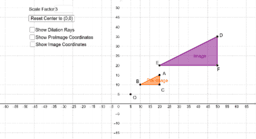# APS Geometry Unit 3 Book

Topic:
Geometry
Understand similarity in terms of similarity transformations MGSE9-12.G.SRT.1 Verify experimentally the properties of dilations given by a center and a scale factor. a. The dilation of a line not passing through the center of the dilation results in a parallel line and leaves a line passing through the center unchanged. b. The dilation of a line segment is longer or shorter according to the ratio given by the scale factor. MGSE9-12.G.SRT.2 Given two figures, use the definition of similarity in terms of similarity transformations to decide if they are similar; explain, using similarity transformations, the meaning of similarity for triangles as the equality of all corresponding pairs of angles and the proportionality of all corresponding pairs of sides. MGSE9-12.G.SRT.3 Use the properties of similarity transformations to establish the AA criterion for two triangles to be similar. Prove theorems involving similarity MGSE9-12.G.SRT.4 Prove theorems about triangles. Theorems include: a line parallel to one side of a triangle divides the other two proportionally, (and its converse); the Pythagorean Theorem using triangle similarity. MGSE9-12.G.SRT.5 Use congruence and similarity criteria for triangles to solve problems and to prove relationships in geometric figures. Understand and apply theorems about circles MGSE9-12.G.C.1 Understand that all circles are similar.